# CRCT Review Wednesday February 17 2010 2 Algebraic

• Slides: 7
Download presentationCRCT Review Wednesday February 17, 2010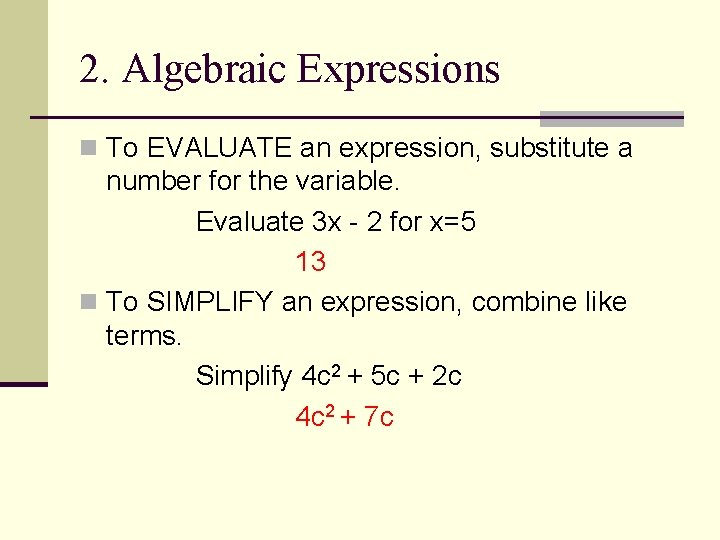2. Algebraic Expressions n To EVALUATE an expression, substitute a number for the variable. Evaluate 3 x - 2 for x=5 13 n To SIMPLIFY an expression, combine like terms. Simplify 4 c 2 + 5 c + 2 c 4 c 2 + 7 c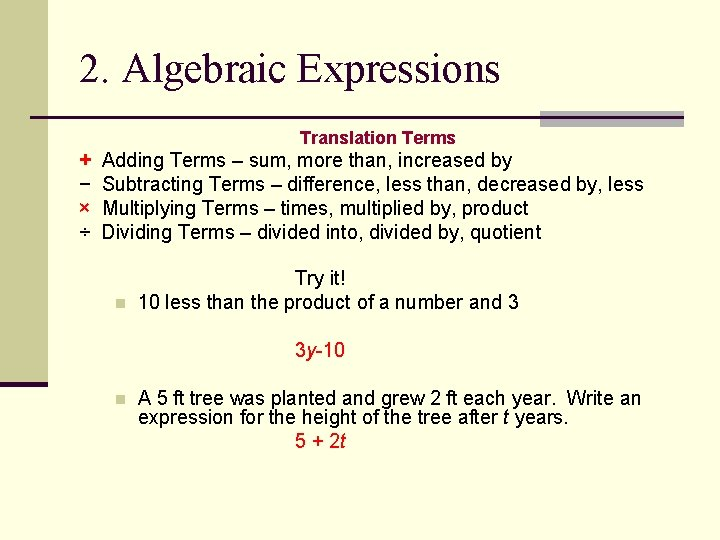2. Algebraic Expressions Translation Terms + − × ÷ Adding Terms – sum, more than, increased by Subtracting Terms – difference, less than, decreased by, less Multiplying Terms – times, multiplied by, product Dividing Terms – divided into, divided by, quotient n Try it! 10 less than the product of a number and 3 3 y-10 n A 5 ft tree was planted and grew 2 ft each year. Write an expression for the height of the tree after t years. 5 + 2 t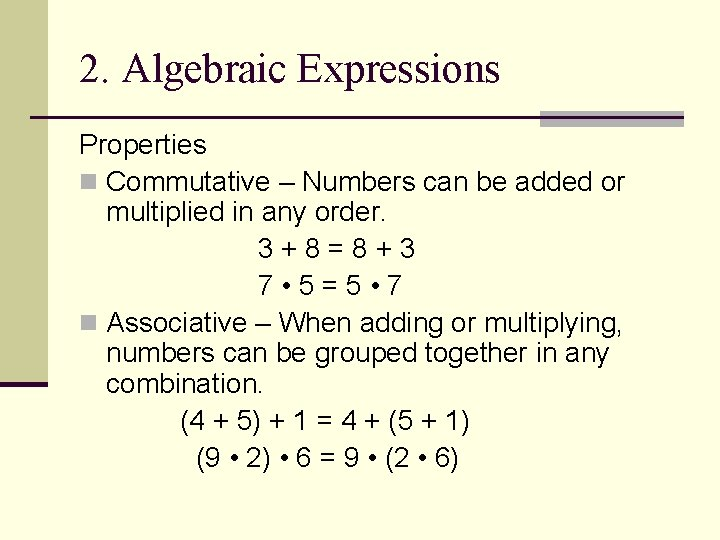2. Algebraic Expressions Properties n Commutative – Numbers can be added or multiplied in any order. 3+8=8+3 7 • 5=5 • 7 n Associative – When adding or multiplying, numbers can be grouped together in any combination. (4 + 5) + 1 = 4 + (5 + 1) (9 • 2) • 6 = 9 • (2 • 6)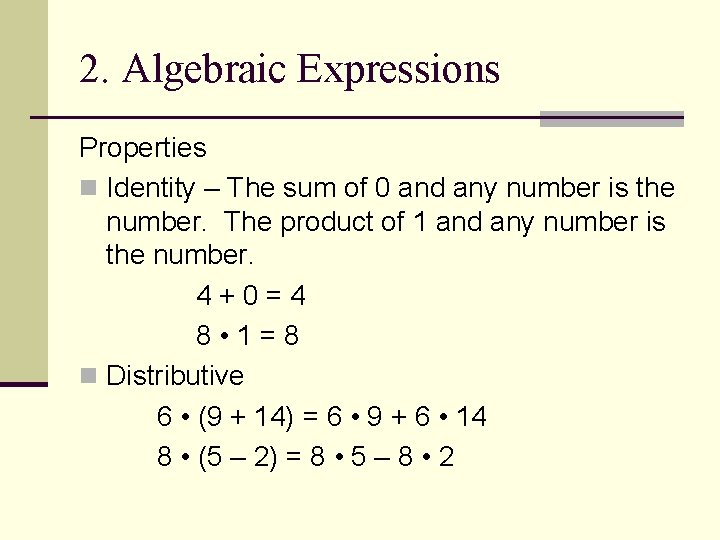2. Algebraic Expressions Properties n Identity – The sum of 0 and any number is the number. The product of 1 and any number is the number. 4+0=4 8 • 1=8 n Distributive 6 • (9 + 14) = 6 • 9 + 6 • 14 8 • (5 – 2) = 8 • 5 – 8 • 22. Algebraic Expressions Don’t forget about PEMDAS!!!!! n Parentheses n Exponents n Multiplication n Division n Addition n Subtraction (32 + 11) ÷ 5 4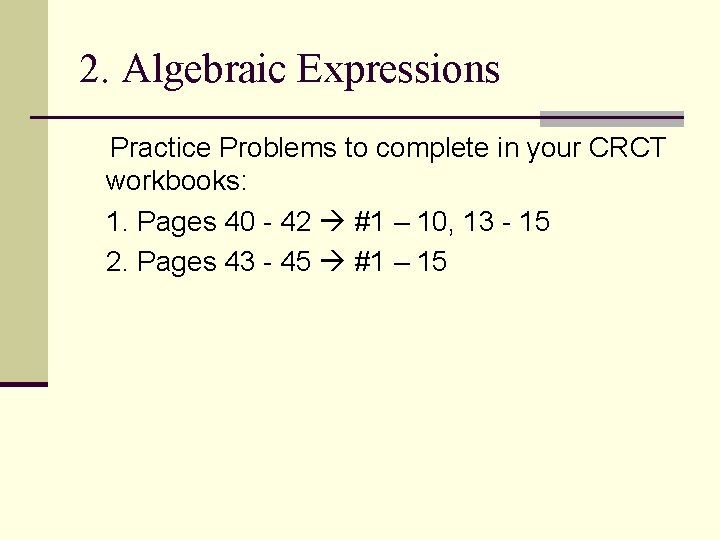2. Algebraic Expressions Practice Problems to complete in your CRCT workbooks: 1. Pages 40 - 42 #1 – 10, 13 - 15 2. Pages 43 - 45 #1 – 15